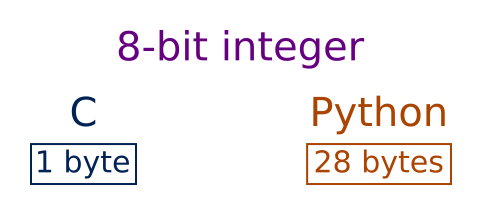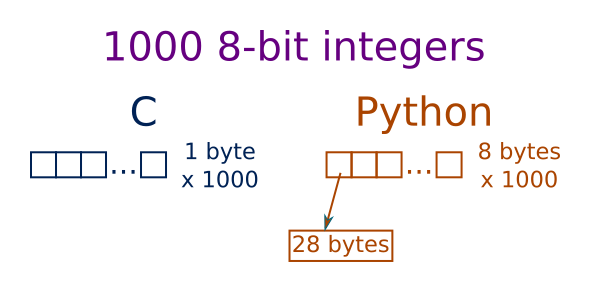# Numpy efficiency

Martin McBride, 2017-05-10
Tags arrays, efficiency
Categories numpy
In section Python librariesWhen we talk about the efficiency of numpy arrays, what exactly do we mean? There are really two separate issues:

• Efficiency of memory usage.
• Processing efficiency.

## Memory usage

Numpy is written in C, which is quite a low level language. Values are stored directly in memory. If you want to store an 8-bit integer using C, it takes exactly one byte.

In Python, numbers are stored as objects. Every Python object has to store information about its type, and also a reference count (to tell Python when the object is no longer being used, so it can be automatically deleted), in addition to the actual value of the object. The exact amount of memory used can depend on the type of computer you have (whether it is a 32-bit or 64-bit system). On my 64 bit computer, it takes 28 bytes to store an 8-bit integer.Now consider an array of 1,000 8-bit numbers. In C, the elements of an array are stored one after the other in a single block of memory. So an array of 1000 8-bit numbers takes exactly 1,000 bytes.

In Python, a list is an object, and each of its elements (the numbers) is another separate object. The list contains an array of references, which point to the element objects. On a 64-bit computer, each reference is 8 bytes long. With various other data the list has to store, the list object itself is a little over 8,000 bytes.

But don't forget, that is just the list! Each element of the list (each number) requires 28 bytes. So 1,000 elements take over 36,000 bytes of storage.If you have a 2 dimensional array of 1000 by 1000 elements, again C stores these elements on after the other in a single block of memory. It stores the 1000 bytes of the first row, followed immediately by the 1000 bytes of the second row, etc. So the whole arrays takes exactly 1,000,000 bytes (1,000 x 1,000).

In Python, a 2D list is implemented as a list of lists. Each row is a separate list of 1000 elements (taking about 36,000 bytes). There are 1,000 of these lists, one for each row. There is also the main list, the list of all the rows - but with so many lists already, one extra doesn't make much difference to the total amount of space used.

## Example of memory usage

A high quality digital image of about 10 million pixels would require about 30 MBytes to store in a numpy array, but would take about a gigabyte to store as a standard Python list.

If you were writing an image editor, you might need to store several versions of the image in memory (for example, if you wanted to be able to undo the most recent changes), which would take several gigabytes. If you wanted to edit several images at the same time, you would need tens of gigabytes, which starts to get unmanageable. Using numpy arrays requires a fraction of the memory.

## Processing efficiency

Suppose we wanted to take an existing numpy array a, and use it to create a new numpy array b, where each element of b is one greater than the corresponding element of a. That is, if a is:

[1, 3, 8, 0]


we would create b with elements:

[2, 4, 9, 1]


The code to do this is (assuming a contains a numpy array):

b = a + 1


The whole process of looping over the values in a, adding 1 to each value, and storing the result in b, is executed in highly efficient code in the numpy library. Here is a diagram of what happens:Arrays a and b are stored contiguously in separate areas of memory. A pointer p1 points to the current element in a, and p2 points to the current element in b. Here is what the main loop does (assuming n is the length of a):

1. Loop n times:
2. Read the value from memory location p1
3. Add 1 to the value
4. Stote the new value in memory location p2
5. Increment p1
6. Increment p2

Each of these operations is very simple, and might be performed by a single CPU instruction. The whole loop might take just a handful of instructions, and run very quickly indeed.

Now let's do the same thing with normal Python lists. The code would look like this:

b = []
for x in a:
b.append(x+1)


or if you prefer to use list comprehensions:

b = [x + 1 for x in a]


Here is what is python needs to do to execute this loop:

    1. Create an iterator to loop over a:
2.   Get the next value of a, as x
3.   Calculate x + 1 and create a new number object
4.   Append the value to bThis code isn't horribly inefficient, it will still run quite quickly. Python itself may optimise some of these steps. But it will be a lot slower than the highly optimised code in the numpy library, for several reasons:

• It uses an iterator to get the values from a, rather than a simple pointer.
• Values in the list are Python int objects, rather than simple byte values.
• Numbers in Python are immutable, so in order to add 1 to x a new number object must be created.
• The result has to be appended to list b rather than just written to a memory location.

This might take a few dozen instructions, rather than a handful that numpy takes, and might run 10 times slower (of course, a lot depends on exactly what you are doing, and what type of processor you are running on, and how much optimisation Python can perform).

Visit the PythonInformer Discussion Forum for numeric Python.

If you found this article useful, you might be interested in the book NumPy Recipes, or other books, by the same author.

<<Prev

#### Popular tags

2d arrays abstract data type alignment and animation arc array arrays bezier curve built-in function circle close closure colour comparison operator comprehension context conversion data types design pattern device space dictionary drawing duck typing efficiency encryption enumerate fill filter font font style for loop function function composition function plot functools game development generativepy tutorial generator geometry gif gradient greyscale higher order function html image processing imagesurface immutable object index inner function input installing iter iterator itertools koch curve l system lambda function len line linspace list list comprehension logical operator lru_cache mandelbrot map monad mutability named parameter numeric python numpy object open operator optional parameter or partial application path polygon positional parameter print pure function pycairo radial gradient range recipes rectangle recursion reduce rgb rotation scaling sector segment sequence slice slicing sound spirograph sprite square str stream string stroke subpath symmetric encryption template text text metrics transform translation transparency tuple turtle unpacking user space vectorisation webserver website while loop zip

Copyright (c) Axlesoft Ltd 2020